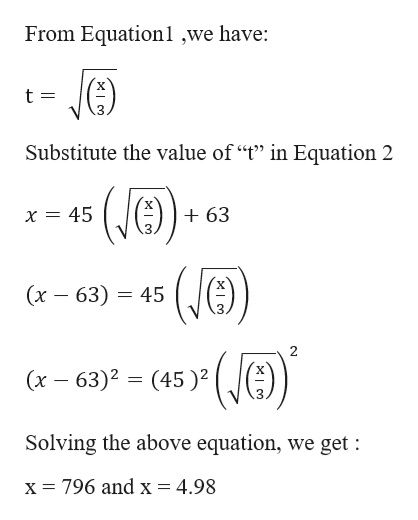Solve the following two equations for the (positive) time, t, and the position, x. Assume SI units.x = 3.00t2andx = 45.0t + 63.0(b) the position, xm

Question

Solve the following two equations for the (positive) time, t, and the position, x. Assume SI units.

x = 3.00t2
and
x = 45.0t + 63.0
(b) the position, x
m
Step 1

Given Information :
x = 3t2 -----Equation1
x ...help_outlineImage TranscriptioncloseFrom Equationl ,we have: t= Substitute the value of "t" in Equation 2 (e) (C (JE) x 45 +63 (х — 63) — 45 (x 63)2 (45) Solving the above equation, we get : x= 796 and x = 4.98 fullscreen

Want to see the full answer?

See Solution

Want to see this answer and more?

Our solutions are written by experts, many with advanced degrees, and available 24/7

See Solution
Tagged in

Kinematics